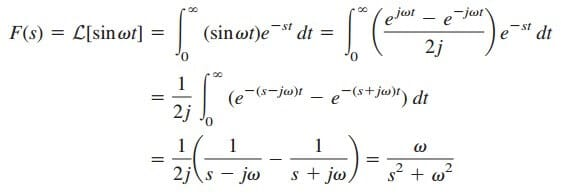# Learn Laplace Transform Definition EasilyBefore start using this method, we need to know what is the Laplace transform definition first.

Contents

Knowing its definition, equation, and condition will help us a lot in implementing this Laplace transform method.

## Laplace Transform Definition

Given a function ƒ(t), its Laplace transform, denoted by F(s) or L[f(t)],
is given by

where s is a complex variable given by

Since the argument st of the exponent e in Equation.(1) must be dimensionless, it follows that s has the dimensions of frequency and units of inverse seconds (s1).

In Equation.(1), the lower limit is specified as 0to indicate a time just before t = 0. We use 0 as the lower limit to include the origin and capture any discontinuity of f(t) at t = 0; this will accommodate functions—such as singularity functions—that may be discontinuous at t = 0.

For an ordinary function f(t), the lower limit can be replaced by 0.

The Laplace transform is an integral transformation of a function f(t) from the time domain into the complex frequency domain, giving F(s).

We assume in Equation.(1) that f(t) is ignored for t < 0. To ensure that this is the case, a function is often multiplied by the unit step. Thus, f(t) is written as f(t)u(t) or f(t), t 0.

The Laplace transform in Equation.(1) is known as the one-sided (or unilateral) Laplace transform. The two-sided (or bilateral) Laplace transform is given by

The one-sided Laplace transform in Equation.(1), being adequate for our purposes, is the only type of Laplace transform that we will treat in this book.

A function f(t) may not have a Laplace transform. In order for f(t) to have a Laplace transform, the integral in Equation.(1) must converge to a finite value. Since |ejωt| = 1 for any value of t, the integral converges when

for some real value σ = σc. Thus, the region of convergence for the Laplace transform is Re(s) = σ > σc, as shown in Figure.(1). In this region, |F(s)| < and F(s) exists. F(s) is undefined outside the region of convergence.

Fortunately, all functions of interest in circuit analysis satisfy the convergence criterion in Equation.(4) and have Laplace transforms.

Therefore, it is not necessary to specify σc in what follows.

A companion to the direct Laplace transform in Equation.(1) is the inverse Laplace transform given by

where the integration is performed along a straight line (σ1 + , -∞ < ω < ) in the region of convergence, σ1 > σc. See Figure.(1). The direct application of Equation.(5) involves some knowledge about complex analysis beyond the scope of this book.

For this reason, we will not use Equation.(5) to find the inverse Laplace transform. The functions f(t) and F(s) are regarded as a Laplace transform pair where

meaning that there is a one-to-one correspondence between f(t) and F(s). The following examples derive the Laplace transforms of some important functions.

## Laplace Transform Definition Examples

Below is the laplace transform definition examples:

### Laplace Transform Definition Example 1

Determine the Laplace transform of each of the following functions: (a) u(t), (b) eatu(t), a 0, and (c) δ(t).

Solution:

(a) For the unit step function u(t), shown in Figure.(2a), the Laplace transform is

(b) For the exponential function, shown in Figure.(2b), the Laplace transform is

(c) For the unit impulse function, shown in Figure.(2c),